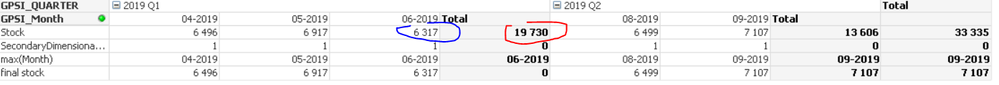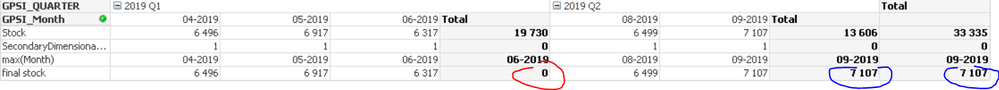# QlikView App Development

Discussion Board for collaboration related to QlikView App Development.

Announcements
Modernize Your QlikView Deployment webinar, Nov. 3rd. REGISTER
cancel
Showing results for
Did you mean:
HighlightedContributor II

## changing calculation for Partial Sums using SecondaryDimentionality

Hello Colleagues,

I need to change the logic of partial sums calculation in pivot table. For year quartes, instead of sum of 3 month, I need the last month value to be presented as quarter total. I use SecondaryDimentionality but it won't work properly'

In given example, instead of 19730 (sum of months) I want to get 6317 (last month val)To solve this I use secondary dimentionality to locate on which level of cumulation given section is.

After that I use Max(Month) to check if last month of the quarter is properly defined for quarter total column  - and it works - for Q1 I get 06-2019, for Q2 I get 09-2019.

then I use Max(Month) in final formula:

if(
SecondaryDimensionality() = 1,
sum(Stock),
sum( {<Month = {'\$(=Max(Month))'}>} Stock)
)

Sadly the result is correct only for highest quarter . In example below - correct for Q2 and total overall, incorrect for Q1. It's strange because Max(Month) correctly assigned 06-2019 as last month of Q1.Any ideas where I went wrong?

Best Regards,

Emil

1 Solution

Accepted Solutions
HighlightedMVP

Try doing this using Aggr() function...

``````If(
SecondaryDimensionality() = 1,
Sum(Stock),
Sum(Aggr(If(GPSI_Month = Max(TOTAL <GPSI_QUARTER> GPSI_Month), Sum(Stock)), GPSI_QUARTER, GPSI_Month))
)``````

You might be able to use FirstSortedValue() function, but I would need a sample to test it out.

2 Replies
HighlightedMVP

Try doing this using Aggr() function...

``````If(
SecondaryDimensionality() = 1,
Sum(Stock),
Sum(Aggr(If(GPSI_Month = Max(TOTAL <GPSI_QUARTER> GPSI_Month), Sum(Stock)), GPSI_QUARTER, GPSI_Month))
)``````

You might be able to use FirstSortedValue() function, but I would need a sample to test it out.

HighlightedContributor II

Thank you very much Sunny, this works perfectly!